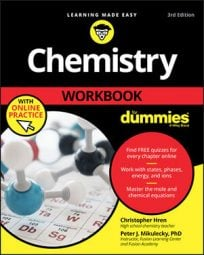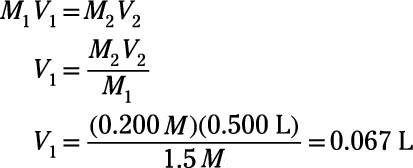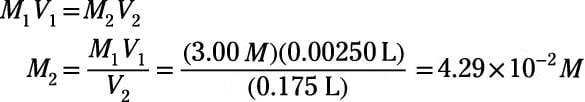##### Chemistry Workbook For Dummies with Online PracticeReal-life chemists in real-life labs don't make every solution from scratch. Instead, they make concentrated stock solutions and then make dilutions of those stocks as necessary for a given experiment.

To make a dilution, you simply add a small quantity of a concentrated stock solution to an amount of pure solvent. The resulting solution contains the amount of solute originally taken from the stock solution but disperses that solute throughout a greater volume. Therefore, the final concentration is lower; the final solution is less concentrated and more dilute.

How do you know how much of the stock solution to use and how much of the pure solvent to use? It depends on the concentration of the stock and on the concentration and volume of the final solution you want. You can answer these kinds of pressing questions by using the dilution equation, which relates concentration (C) and volume (V) between initial and final states:

C1V1 = C2V2
You can use the dilution equation with any units of concentration, provided you use the same units throughout the calculation. Because molarity is such a common way to express concentration, the dilution equation is sometimes expressed in the following way, where M1 and M2 refer to the initial and final molarity, respectively:
M1V1 = M2V2
For example, how would you prepare 500. mL of 0.200 M NaOH(aq) from a stock solution of 1.5 M NaOH?

Start by using the dilution equation,

M1V1 = M2V2
The initial molarity, M1, comes from the stock solution and is therefore 1.5 M. The final molarity is the one you want in your final solution, which is 0.200 M. The final volume is the one you want for your final solution, 500. mL, which is equivalent to 0.500 L. Using these known values, you can calculate the initial volume, V1:The calculated volume is equivalent to 67 mL. The final volume of the aqueous solution is to be 500 mL, and 67 mL of this volume comes from the stock solution. The remainder, 500 mL – 67 mL = 433 mL, comes from pure solvent (water, in this case). So to prepare the solution, add 67 mL of 1.5 M stock solution to 433 mL water. Mix and enjoy (...though not by drinking it, please)!

Try another problem: What is the final concentration in molarity of a solution prepared by diluting 2.50 mL of 3.00 M KCl(aq) up to 0.175 L final volume?

You can use the dilution equation,

M1V1 = M2V2
In this problem, the initial molarity is 3.00 M, the initial volume is 2.50 mL or 2.50 x 10–3 L and the final volume is 0.175 L. Use these known values to calculate the final molarity, M2:So, the final concentration in molarity of the solution is

4.29 x 10–2 M